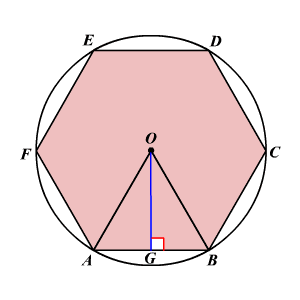Areas of Regular Polygons

Apothem

The apothem of a regular polygon is a segment drawn the center of the polygon (that is, the center of the circle which circumscribes the polygon) to one side, such that it is perpendicular to the side.

Consider the regular hexagon $ABCDEF$ inscribed in a circle $O$ , $\stackrel{¯}{OA}$ and $\stackrel{¯}{OB}$ are radii from the center of the circle $O$ to the two vertices of the hexagon. $\stackrel{¯}{OG}$ is drawn from the center of the regular polygon perpendicular to the side of the polygon. So, $\stackrel{¯}{OG}$ is an apothem.Area of a Regular Polygon

If a regular polygon has an area of $A$ square units, a perimeter of $P$ units, an apothem of $a$ units, then the area is one-half the product of the perimeter and the apothem.

$A=\frac{1}{2}Pa$

Be careful with this formula, though: for a given apothem, there is only ONE POSSIBLE value of $P$ . (You can actually calculate $P$ , but it takes some work.)

Example:

For a regular hexagon with apothem $5$ m, the side length is about $5.77$ m.

Use the formula

$A=\frac{1}{2}Pa$

to find the area of the hexagon.

The perimeter of the hexagon is about $6\left(5.77\right)$ or $34.62$ m.

Now substitute the values.

$A\approx \frac{1}{2}\left(34.62\right)\left(5\right)$

Simplify.

$\begin{array}{l}A\approx \frac{1}{2}\left(173.1\right)\\ \text{\hspace{0.17em}}\text{\hspace{0.17em}}\text{\hspace{0.17em}}\text{\hspace{0.17em}}\text{\hspace{0.17em}}\approx 86.5\end{array}$

Therefore, the area of the regular hexagon is about .